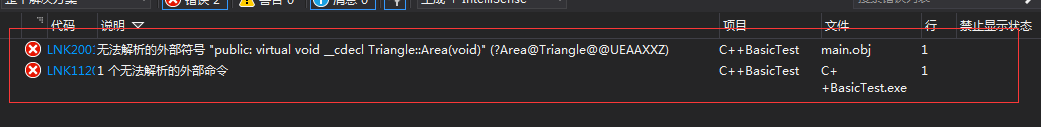C++11 中的 override 关键字，可以显式的在派生类中声明，基类的哪些函数需要被重写，如果没被重写，则编译器会报错。防止出现在需要调用派生类某个成员函数的情况下错误的调用了基类的缺省函数实现。

# 1 派生类对基类中的函数继承

• 接口继承，通过在基类中声明纯虚函数实现
• 接口+缺省实现，通过在基类中声明普通虚函数实现，派生类可以声明同名的虚函数覆盖基类中的缺省实现，以实现自己的个性化
• 接口+强制实现，通过在基类中声明一个非虚函数实现

## 1.1 接口继承通常用不到override关键字

#include <iostream>
#include <memory>

using namespace std;

class Shape
{
public:
virtual void Draw() = 0;
};

class Triangle : public Shape
{
public:
virtual void Draw()
{
std::cout << "Triangle Draw" << std::endl;
}
};

int main()
{
std::shared_ptr<Triangle> pTriangle = std::make_shared<Triangle>();

pTriangle->Draw();

getchar();

return 0;
}


## 1.2 接口+强制实现通常用不到override关键字

#include <iostream>
#include <memory>

using namespace std;

class Shape
{
public:
virtual void Draw() = 0;

void Length()
{
std::cout << "Shape Length" << std::endl;
}
};

class Triangle : public Shape
{
public:
virtual void Draw()
{
std::cout << "Triangle Draw" << std::endl;
}
};

int main()
{
std::shared_ptr<Triangle> pTriangle = std::make_shared<Triangle>();

pTriangle->Draw();

pTriangle->Length();

getchar();

return 0;
}



Triangle Draw
Shape Length


#include <iostream>
#include <memory>

using namespace std;

class Shape
{
public:
virtual void Draw() = 0;

void Length()
{
std::cout << "Shape Length" << std::endl;
}
};

class Triangle : public Shape
{
public:
virtual void Draw()
{
std::cout << "Triangle Draw" << std::endl;
}

void Length()
{
std::cout << "Triangle Length" << std::endl;
}
};

int main()
{
std::shared_ptr<Triangle> pTriangle = std::make_shared<Triangle>();

pTriangle->Draw();

pTriangle->Length();

getchar();

return 0;
}



Triangle Draw
Triangle Length


## 1.3 override关键字通常在派生类对基类普通虚函数的继承上使用

#include <iostream>
#include <memory>

using namespace std;

class Shape
{
public:
virtual void Area()
{
std::cout << "Shape Area" << std::endl;
}
};

class Triangle : public Shape
{
public:

};

int main()
{
std::shared_ptr<Triangle> pTriangle = std::make_shared<Triangle>();

pTriangle->Area();

getchar();

return 0;
}


#include <iostream>
#include <memory>

using namespace std;

class Shape
{
public:
virtual void Area()
{
std::cout << "Shape Area" << std::endl;
}
};

class Triangle : public Shape
{
public:
virtual void Area() override;
};

int main()
{
std::shared_ptr<Triangle> pTriangle = std::make_shared<Triangle>();

pTriangle->Area();

getchar();

return 0;
}#include <iostream>
#include <memory>

using namespace std;

class Shape
{
public:
virtual void Area()
{
std::cout << "Shape Area" << std::endl;
}
};

class Triangle : public Shape
{
public:
virtual void Area() override
{
std::cout << "Triangle Area" << std::endl;
}
};

int main()
{
std::shared_ptr<Triangle> pTriangle = std::make_shared<Triangle>();

pTriangle->Area();

getchar();

return 0;
}



# 2 结论

• 基类中使用纯虚函数，则派生类则是从基类继承接口，并且必须在类中对该接口进行实现
• 基类中使用普通虚函数，则派生类是从基类继承接口和缺省实现，如果派生类重写该接口，则使用派生类的实现，如果没有，则使用基类的缺省实现，而override常使用在此情况中，最好建议在派生类中需要重写的函数声明之后增加关键字override，避免出现逻辑错误
• 基类中使用普通函数，则派生类是从基类继承接口和强制实现，不建议修改继承自基类的非虚函数支付宝扫一扫微信扫一扫纵使晴明无雨色，入云深处亦沾衣。